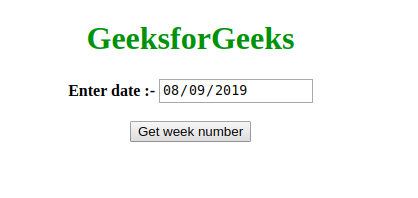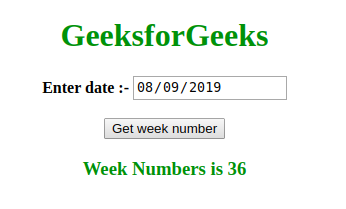Open In App

# How to get the current weeknumber of the year?

The problem is to find the week number of year of a given date (in dd/mm/yy format).

```Input: date = "02/01/2019"
Output: 1
Explanation: We can check from the calendar that 2nd January 2019 lies
in 1st week of 2019.

Input: date = "08/09/2019"
Output: 36
```

Approach:
We will assign each day of the week a number. Let’s say Sunday is assigned 1 and Monday is assigned 2 and so on we assign numbers to other days. According to our convention, each week starts with Sunday and ends on Saturday.
So, suppose today is Monday and hence, the week number is also 1. After 20 days, the week number will be obviously 3rd.

`20 days = 7+7+6 days`

On carefully observing, we can see that week number after p days can be calculated using the formula mentioned below.

```Week Number = least integer [ p/7 ]
```

(Least integer value of x is the smallest integer greater than or equal to x. For example, the Least integer value of 8.9 is 9 and that of 8 is 8).

We can use the above concept to find the week number of a given date as follows:

• Find the day number of 1st January of given year. Let’s call it x.
• Now, find the number of days before given date in given year. Let’s call it y.
• We can calculate the week number using the formula below
`Week Number = least integer [ (x+y)/7 ]`

Example: Below is the implementation of above approach.

 ```<``html``>`` ` `<``head``>``    ``<``title``>Calculate Week Number``    ``<``script``>``        ``Date.prototype.getWeekNumber = function() {`` ` `            ``var oneJan = ``                ``new Date(this.getFullYear(), 0, 1);`` ` `            ``// calculating number of days ``            ``//in given year before given date`` ` `            ``var numberOfDays = ``                ``Math.floor((this - oneJan) / (24 * 60 * 60 * 1000));`` ` `            ``// adding 1 since this.getDay()``            ``//returns value starting from 0`` ` `            ``return Math.ceil((this.getDay() + 1 + numberOfDays) / 7);`` ` `        ``}`` ` `        ``function printWeekNumber() {``            ``var dateInput = ``                ``document.getElementById("dateInput").value;``            ``var date = new Date(dateInput);``            ``var result = date.getWeekNumber();``            ``document.getElementById("result").innerHTML = ``              ``"Week Numbers is " + result;``        ``}``    `````` ` `<``body``>`` ` `    ``<``h1` `style``=``"color: green"` `align``=``"center"``> ``            ``GeeksforGeeks ``        ```` ` `    ``<``p` `align``=``"center"``> <``b``> Enter date :- ``        ``<``input` `type``=``"date"` `id``=``"dateInput"``>``        ``<``br``>``        ``<``br``>`` ` `        ``<``button` `onclick``=``"printWeekNumber()"``>``          ``Get week number``      ````    ```` ` `    ``<``h3` `style``=``"color:green"` `id``=``"result"` `align``=``"center"``>`` ` ``` ` ``

Output:

• Before clicking the button:• After clicking the button: